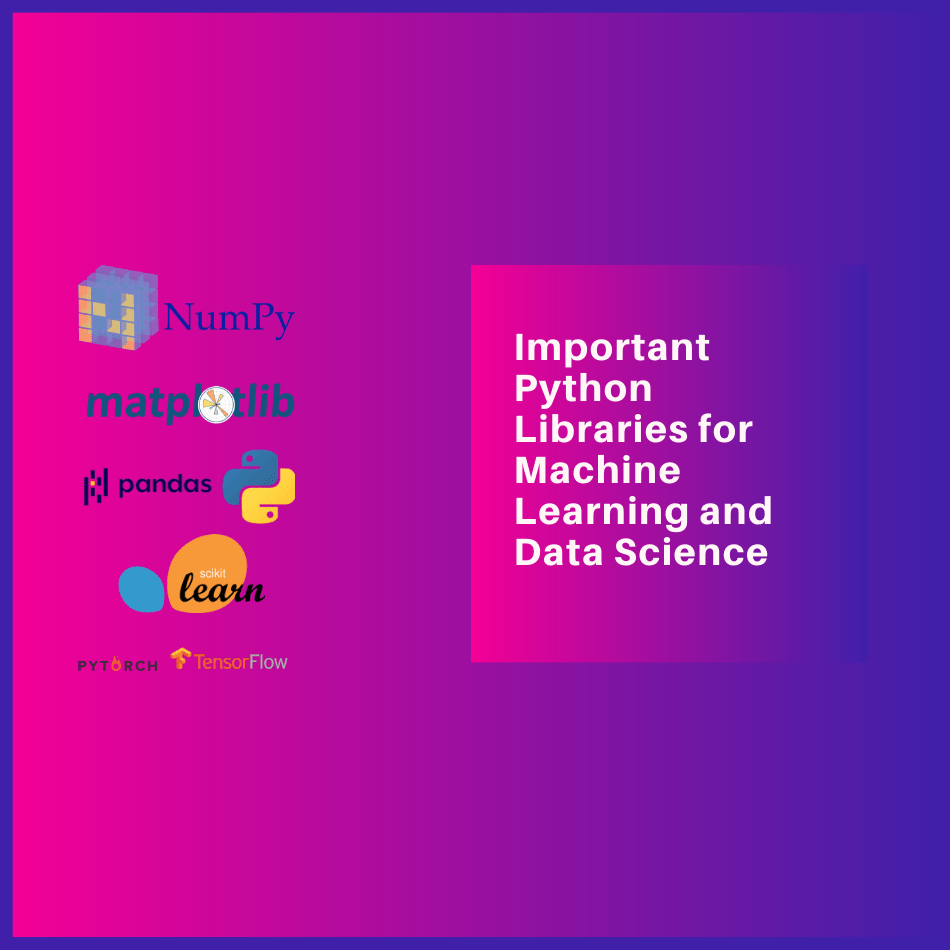In this article, we will mention highly used and vital libraries that you will need while working in Machine learning, Artificial Intelligence and Data Science field with Python as programming language.

Most of machine learning and data science professional prefer Python language because of many libraries and significant support from community.

So, let start with Important Python’s Library for Machine Learning.

I assume you are an enthusiast or have the prior knowledge of Machine Learning and Data Science, you should have an idea about the different phases in machine learning project like data gathering then pre-processing and training-evaluation, etc. I will mention given libraries in order of data gathering and processing(Numpy and Pandas) then for EDA(Matplotlib) and lastly in this article for training and evaluation(TensorFlow, PyTorch and Scikit-learn).

If you do not know the distinct steps involved in machine learning, please read this article first- Click Here.

## NumPy

Numpy and Pandas are the extensively used in machine learning and data science tasks, while diving more into libraries you will get to know a lot of libraries are built on Numpy. So, firstly will talk about Numpy.

NumPy– Numpy is very helpful while working with multi-dimensional array and matrices, it allows us to work with ndarrays and matrices. NumPy is essential for other Python scientific packages like SciPy, scikit-learn and OpenCV.

We use Numpy in multiple places but we use more often during array, matrices and mathematical related tasks, numpy.dot(), numpy.arange(), numpy.squeeze() are few very common methods of numpy.

## Pandas

Pandas– Pandas is another excellent library used for data handling manipulation task. It provides easy-to-use data structures and data analysis, high-performance work, we use it for exploring, cleaning, transforming, visualizing datasets. Pandas is built on the Numpy library.

READ  Supervised Learning: Classification and Regression Problems

You will be using it from reading data from CSV, Excel, API etc to saving data in desired form. Pandas in-built data type DataFrame and Series are broadly used in data science tasks.

## Matplotlib

Matplotlib: Here comes our very favorite visualization library, “Matplotlib is a comprehensive library for creating static, animated, and interactive visualizations in Python” as written in its official library. We use it for creating various kinds of graph and plot to visualize the data.

Using Matplotlib is very easy and anyone can learn in no time, we can generate a graph in just one line of code.

But some time people prefer other libraries for visualizations, another famous visualization library is Seaborn. Seaborn is built on top of matplotlib, it has some easy and often used methods such as Pairplot.

I would like to add one more library, that is Plotly, personally I like Plotly due to its interactive plots and graphs, but obviously, during EDA (Exploratory Data Analysis) you will not need it. Please visit Plotly official page and checkout the impressive features.

## Scikit-learn or sklearn

Scikit-learn or sklearn has methods for your pre-processing, classification, regression, clustering, dimension reduction, etc.

Scikit-learn will fulfil a lot of your machine learning work need, it has method for dividing the data into training and test set; it has various methods to evaluate your trained model such as confusion matrix, ROC curve and much more, most importantly it has numerous machine learning algorithm to train a model.

Lets understand with a simple example for training a model, lets say you want to use SVM(Support Vector Machine) for your classification task, just write `from sklearn import svm` and then `clf = svm.SVC()`, now you are ready with `clf` for the task.

READ  Machine Learning and Machine Learning Algorithms

To be clear, sklearn is not only library for your machine learning algorithms but is one is most popular library for it.

## TensorFlow and PyTorch

TensorFlow and PyTorch are two widely used libraries for deep learning tasks. TensorFlow is developed by Google whereas PyTorch is developed in Facebook. These two libraries make your deep learning tasks easy to implement.

Coming to which one of TensorFlow and PyTorch should be used? People have different opinion about this, some prefer PyTorch and other prefer TensorFlow. Although, if we go by numbers, TensorFlow is used more than PyTorch.

Conclusion:

These are the few libraries are used often in machine learning, deep learning and data science projects, but there are many more libraries that you will encounter while working with diverse requirements.

(All product names, logos, and brands are property of their respective owners.)

This site uses Akismet to reduce spam. Learn how your comment data is processed.